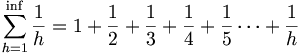# Harmonics$\sum_{h=1}^\inf \frac{1}{h} = 1 + \frac{1}{2} + \frac{1}{3} + \frac{1}{4} + \frac{1}{5} \cdots + \frac{1}{h}$